Courses

# Electron Emission and Its Processes Class 12 Notes | EduRev

## JEE : Electron Emission and Its Processes Class 12 Notes | EduRev

The document Electron Emission and Its Processes Class 12 Notes | EduRev is a part of the JEE Course Physics For JEE.
All you need of JEE at this link: JEE

What is Electron Emission?

• When light is incident on a metal surface it was observed that electrons are ejected from a metal surface some times even when incredibly dim light such as that from stars and distant galaxies incident on it and some time electrons does not come out from the metal surface even high energetic or high-intensity light falling on the metal surface.
• This shows that the electron emission from a metal surface does not depend on the intensity of incident light but it basically depends on the energy of the incident.
• Photons no matters in the number of photons are very less in dim light, photoelectric effect can be seen.
• During the phenomenon of the photoelectric effect, one incident photon on the metal surface can eject at most only one electron.
• A photon is an energy packet that is fully absorbed not partially. Thus one photon can not be absorbed by more than one electron.
• The minimum amount of energy of photon required to eject an electron out of a metal surface is called work function It is denoted by φ.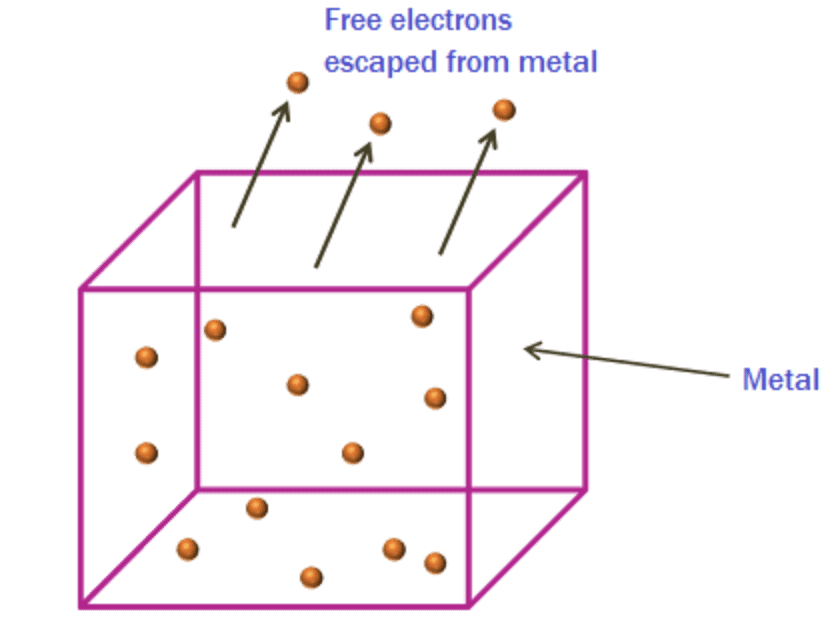However, the surface barrier can be broken by providing a certain minimum amount of energy to the free electrons which increases their kinetic energy and consequently help them escape the metal surface.

This minimum amount of energy is known as the work function of the metal. And when the work function is provided to the metal, the consequent liberation of electrons from the metal surface is known as electron emission.

The work function of a metal depends on:

1. The electron emission from metal only depends on the work function or energy of one photon.
2. But how many electrons come out from the metal is depends on the intensity of the falling light on the energy of the light.
3. The energy of photon incident on metal will not necessarily cause emission of an electron even if its energy is more than work function. The electron after absorption may be involved in many other processes like collision etc in which it can lose energy hence the ratio of no. of electrons emitted to the no. of photons incident on the metal surface is less than unity.

Types of Electron Emission

The electron emission is possible only if sufficient energy (equal to the work function of the metal) is supplied to the metal in the form of heat energy, light energy, etc. Depending on the source of energy, electron emission can be of the following types:

1. Thermionic Emission: In this type, the metal is heated to a sufficient temperature to enable the free electrons to come out of its surface.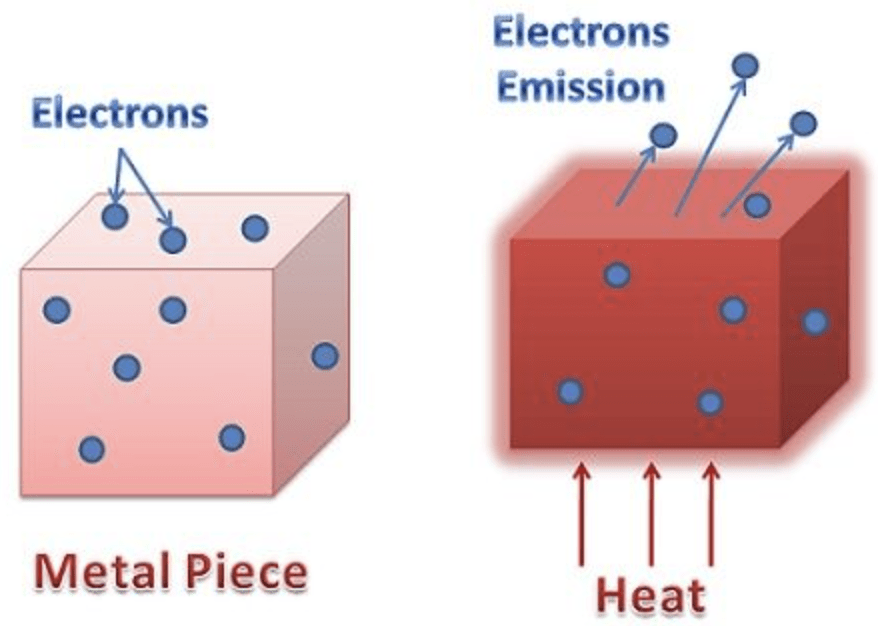2. Field Emission: In this type, a very strong electric field is applied to the metal which pulls the electrons out of the surface due to the attraction of the positive field.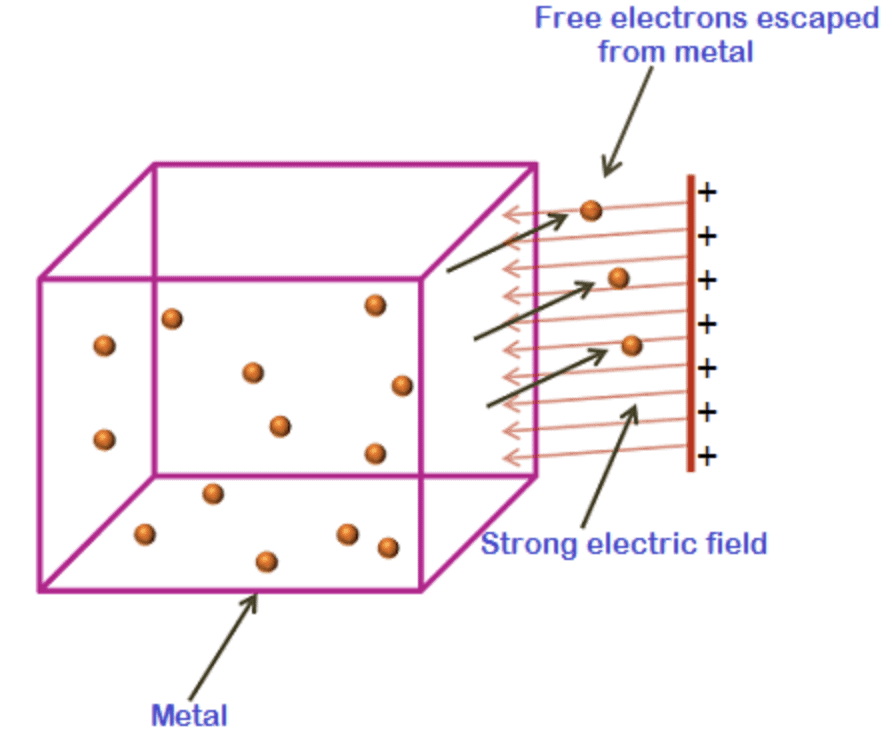3. Photoelectric Emission: In this type, the light of a certain frequency is made to fall on the metal surface which leads to the emission of electrons.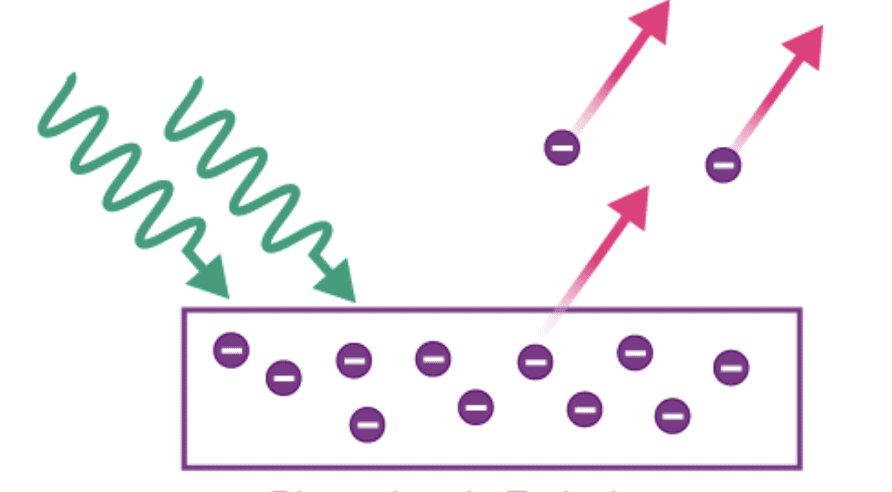Try yourself:Which of the following are quick electron emissions?

Hertz And Lenard's Observations of Photoelectric Effect

• It was in 1887 when Heinrich Hertz was conducting experiments to prove Maxwell’s electromagnetic theory of light, that he noticed a strange phenomenon. Hertz used a spark gap (two sharp electrodes placed at a small distance so that electric sparks can be generated) to detect the presence of electromagnetic waves. To get a closer look, he placed it in a dark box and found that the spark length was reduced. When he used a glass box, the spark length increased and when he replaced it with a quartz box, the spark length increased further. This was the first observation of the photoelectric effect.
• A year later, Wilhelm Hallwachs confirmed these results and showed that UV light on a Zinc plate connected to a battery generated a current (because of electron emission). In 1898, J.J. Thompson found that the amount of current varied with the intensity and frequency of the radiation used.
• In 1902, Lenard observed that the kinetic energy of electrons emitted increased with the frequency of radiation used. This could not be explained as Maxwell’s electromagnetic theory (which Hertz proved correct) predicted that the kinetic energy should be only dependent on light intensity (not frequency).
• The resolution would only come a few years later by Einstein when he would provide an explanation to the photoelectric effect.

Experimental Set-Up

J.J. Thompson’s set-up (later improved by Lenard) to study this effect is of great importance. It consists of two zinc plate electrodes placed on the opposite ends of an evacuated (a vacuum is maintained) glass tube. A small quartz window illuminates one of the electrodes that is made the cathode. Quartz is used because ordinary glass blocks Ultra-Violet light. A variable voltage is exerted across the two electrodes using a battery and a potentiometer. The current in the circuit can be recorded using an ammeter as the potential and light intensity is changed. The set-up is shown below: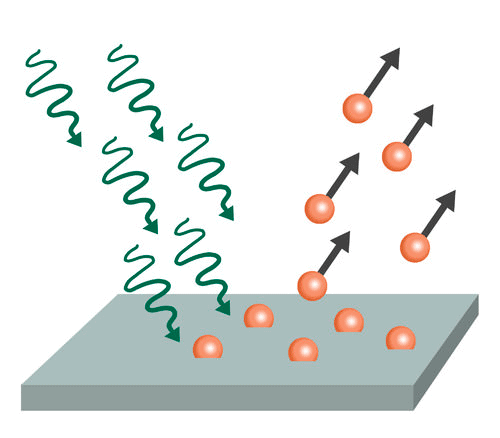Observations

1. The photoelectric current (same as the rate of emission of electrons) is directly proportional to the intensity of light falling on the electrode. Note from the figure below that with increasing intensity the current is increasing. Also, observe that as the voltage has decreased the current also decreases. But to obtain zero current, the voltage has to be reversed to a certain V­0 known as the stopping potential. The voltage must be reversed to such an extent that the electrons cannot reach the anode. This is the maximum kinetic energy an emitted electron can achieve,
Maximum Kinetic energy, KE=eV0
(e is the charge of the electron)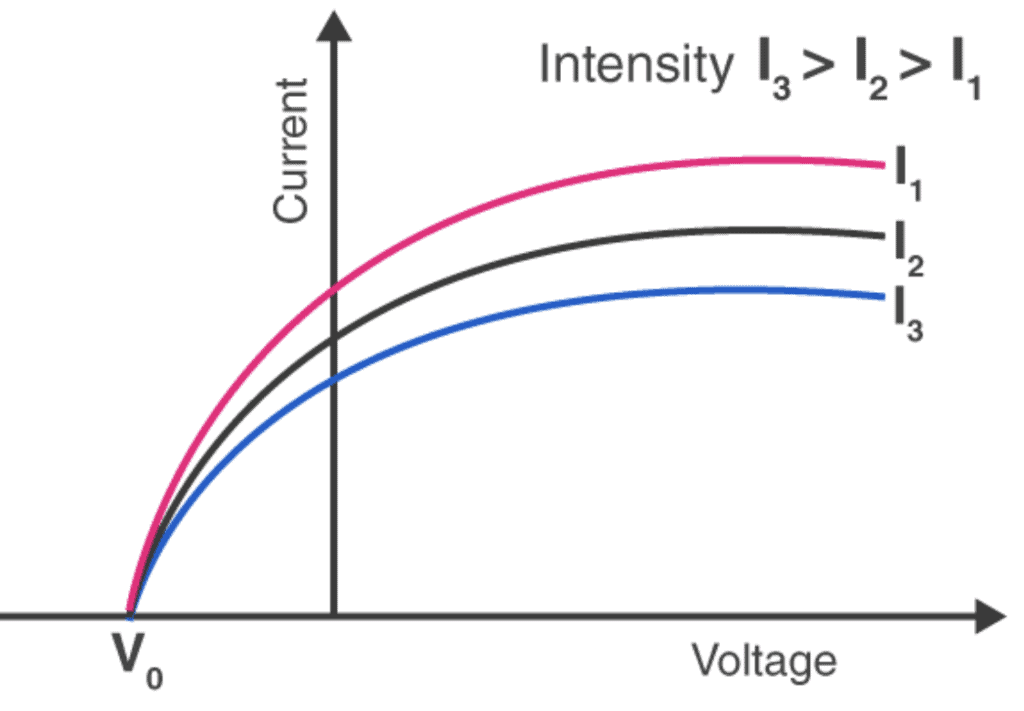Note that the stopping potential is independent of the intensity of light.
2. The Maximum kinetic energy increases with an increase in the frequency of light. With a higher frequency of light (ν), the stopping potential becomes more negative which implies that the kinetic energy of electrons also increases.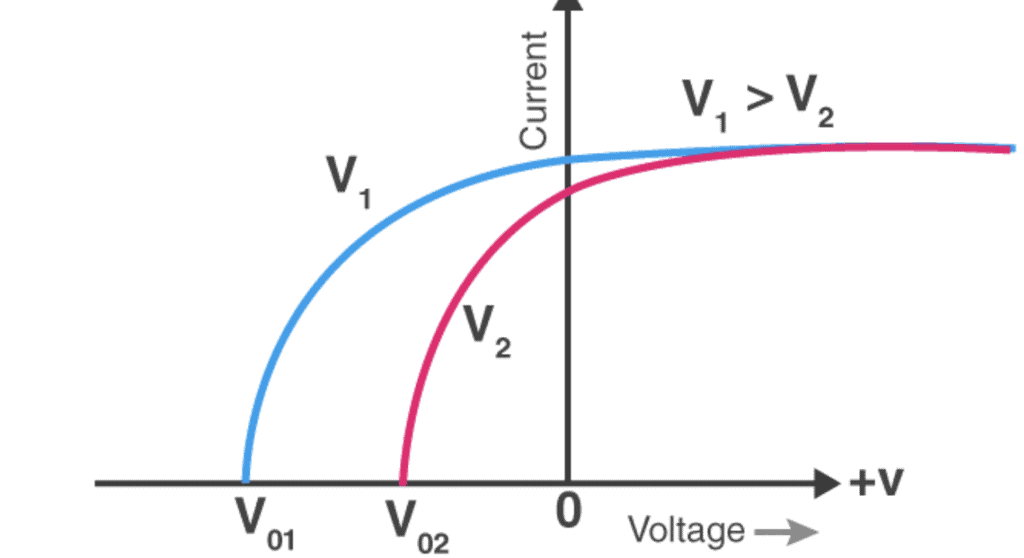3. All frequencies of light, however, cannot cause a photoelectric current to develop. Only light above a certain frequency (ν0) can produce a photoelectric current. This frequency is known as the threshold frequency. This varies with the electrode material. Also, the maximum kinetic energy of the electrons increases linearly with increasing light frequency. If we extend the graph below the x-axis, the intercept on the Kinetic energy axis represents the minimum energy required for emission of the electron; this is known as the work function of the material.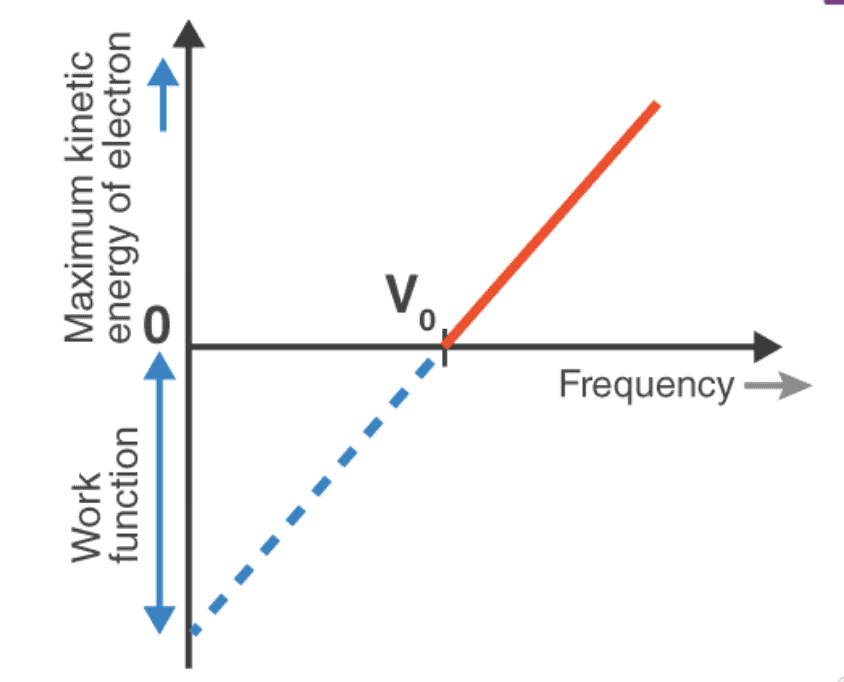4. Lastly, the electron emission occurs instantly without any time lag.

Threshold Frequency and Threshold Wavelength

• We have discussed that to start photoelectric emission, the energy of incident photon on the metal surface must be more than the work function of the metal. If f is the work function of the metal then there must be a minimum frequency of the incident light photon which is just able to eject the electron from the metal surface. This minimum frequency or threshold frequency vth can be given as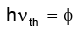• Threshold frequency nth is a characteristic property of metal as it is the minimum frequency of the light radiation required to eject a free electron from the metal surface.
• As the threshold frequency is defined, we can also define the threshold wavelength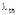for a metal surface. Threshold wavelength is also called cut off wavelength. For a given metal surface threshold wavelength is the longest wavelength at which photoelectric effect is possible. Thus we have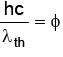• So for the wavelength of incident light λ > λth, the energy of incident photons will become less than the work function of the metal and hence photoelectric effect will not start.
• Thus for a given metal surface photoelectric emission will start at v > vth or λ > λth

Try yourself:Find the wrong statement.

Example 1: The photoelectric threshold of the photoelectric effect of a certain metal is 2750 Å. Find

(i) The work function of emission of an electron from this metal,

(ii) Maximum kinetic energy of these electrons,

(iii) The maximum velocity of the electrons ejected from the metal by light with a wavelength of 1800 Å.

Ans: (i) Given that the threshold wavelength of a metal is λth =  2750 Å. Thus work function of metal can be given as φ =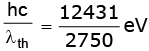(ii) The energy of an incident photon of wavelength 1800 Å on metal in eV is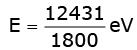= 6.9 eV

Thus maximum kinetic energy of ejected electrons is

KEmax = E – φ = 6.9 - 4.52 eV = 2.38 eV

(iii) If the maximum speed of ejected electrons is vmax then we have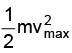= 2.38 eV

or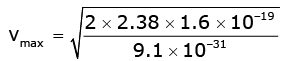= 9.15 X 105 m / s

Example 2: Light quanta with an energy of 4.9 eV eject photoelectrons from metal with work function of 4.5 eV. Find the maximum impulse transmitted to the surface of the metal when each electron flies out.

Ans: According to Einstein's photoelectric equation

E == hv - φ = 4.9 - 4.5 = 0.4 eV

If E be the energy of each ejected photoelectron momentum of electrons is p =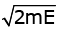We know that change of momentum is impulse. Here the whole momentum of the electron is gained when it is ejected out thus impulse on the surface is Impulse =Substituting the values, we get

Maximum impulse =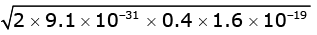= 3.45 X10 -25 kg m / sec

Example 3: In an experiment tungsten cathode which has a threshold of 2300 Å is irradiated by the ultraviolet light of wavelength 1800 Å. Calculate

(i) Maximum energy of emitted photoelectron and

(ii) Work function for tungsten

(Mention both the results in electron-volts)

Given Plank's constant h = 6.6 X 10 -34 joule-sec, 1 eV = 1.6 X 10 -19joule and velocity of light c = 3 X 108 m/sec

Ans: The work function of tungsten cathode is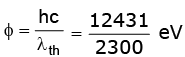= 5.4 eV

The energy in eV of incident photons is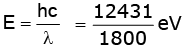The maximum kinetic energy of ejected electrons can be given as

KEmax = E  - φ = 6.9 - 5.4 eV = 1.5 eV

Example 4: Light of wavelength 1800 Å ejects photoelectrons from a plate of a metal whose work functions is 2 eV. If a uniform magnetic field of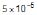tesla is applied parallel to the plate, what would be the radius of the path followed by electrons ejected normally from the plate with maximum energy.

Ans: Energy of incident photons in eV is given as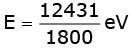As work function of metal is 2 eV, the maximum kinetic energy of ejected electrons is KEmax = E  - φ = 6.9 – eV = 4.9 eV

If vmax be the speed of fasted electrons then we have= 4.9 X1.6 X10 -19 joule

or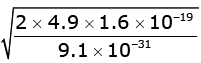= 1.31 X 106 m/s

When an electron with this speed enters a uniform magnetic field normally it follows a circular path whose radius can be given by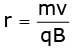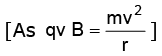or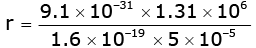or r = 0.149 m

Try yourself:Two metals A and B have work functions 4 eV and 10 eV respectively. Which metal has a higher threshold wavelength?

Wave Theory of Light

• Light always piqued the curiosity of thinkers and scientists. But it wasn’t until the late 17th century that scientists began to comprehend the properties of light. Sir Isaac Newton proposed that light was made of tiny particles known as photons while Christian Huygens believed that light was made of waves propagating perpendicular to the direction of its movement.
• In 1678, Huygens proposed that every point that a luminous disturbance meets turns into a source of the spherical wave itself. The sum of the secondary waves, which are the result of the disturbance, determines what form the new wave will take. This theory of light is known as the ‘Huygens’ Principle’.
• Using the above-stated principle, Huygens was successful in deriving the laws of reflection and refraction of light. He was also successful in explaining the linear and spherical propagation of light using this theory. However, he wasn’t able to explain the diffraction effects of light. Later, in 1803, the experiment conducted by Thomas Young on the interference of light proved the Huygens wave theory of light to be correct. Later in 1815, Fresnel provided mathematical equations for Young’s experiment.
• Max Planck proposed that light is made of finite packets of energy known as a light quantum and it depends on the frequency and velocity of light. Later, in 1905, Einstein proposed that light possessed the characteristics of both particle and wave. He suggested that light is made of small particles called photons. Quantum mechanics gave proof of the dual nature of light.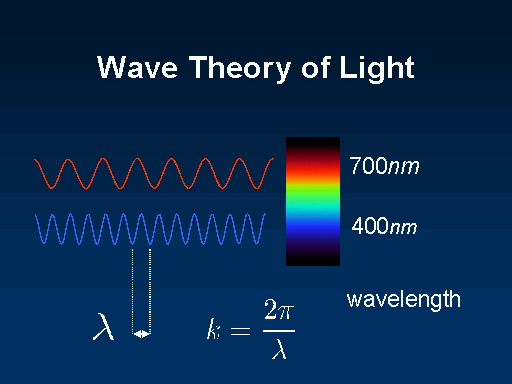Features of the Photoelectric effect cannot be explained in terms of the classical wave theory of light.
1. The intensity problem: Wave theory requires that the oscillating electric field vector E of the light wave increases in amplitude as the intensity of the light beam is increased. Since the force applied to the electron is eE, this suggests that the kinetic energy of the photoelectrons should also be increased the light beam is made more intense. However, observation shows that maximum kinetic energy is independent of the light intensity.
2. The frequency problem: According to the wave theory, the photoelectric effect should occur for any frequency of the light, provided only that the light is intense enough to supply the energy needed to eject the photoelectron. However observations show that there exists for each surface a characteristic cutoff frequency nth, for frequency less than nth, the photoelectric effect does not occur, no matter how intense is light beam.
3. The time delay problem: If the energy acquired by a photoelectron is absorbed directly from the wave incident on the metal plate, the "effective target area" for an electron in the metal is limited and probably not much more than that of a circle of diameter roughly equal to that of an atom. In classical theory, the light energy is uniformly distributed over the wavefront. Thus, if the light is feeble enough, there should be a measurable time lag, between the impinging of the light on the surface and the ejection of the photoelectron. During this interval, the electron should be absorbing energy from the beam until it had accumulated enough to escape. However, no detectable time lag has ever been measured.
Offer running on EduRev: Apply code STAYHOME200 to get INR 200 off on our premium plan EduRev Infinity!

## Physics For JEE

188 videos|492 docs|265 tests

,

,

,

,

,

,

,

,

,

,

,

,

,

,

,

,

,

,

,

,

,

;